Test: Control Systems - 1

# Test: Control Systems - 1 - Electrical Engineering (EE)

Test Description

## 25 Questions MCQ Test Electrical Engineering SSC JE (Technical) - Test: Control Systems - 1

Test: Control Systems - 1 for Electrical Engineering (EE) 2023 is part of Electrical Engineering SSC JE (Technical) preparation. The Test: Control Systems - 1 questions and answers have been prepared according to the Electrical Engineering (EE) exam syllabus.The Test: Control Systems - 1 MCQs are made for Electrical Engineering (EE) 2023 Exam. Find important definitions, questions, notes, meanings, examples, exercises, MCQs and online tests for Test: Control Systems - 1 below.
Solutions of Test: Control Systems - 1 questions in English are available as part of our Electrical Engineering SSC JE (Technical) for Electrical Engineering (EE) & Test: Control Systems - 1 solutions in Hindi for Electrical Engineering SSC JE (Technical) course. Download more important topics, notes, lectures and mock test series for Electrical Engineering (EE) Exam by signing up for free. Attempt Test: Control Systems - 1 | 25 questions in 50 minutes | Mock test for Electrical Engineering (EE) preparation | Free important questions MCQ to study Electrical Engineering SSC JE (Technical) for Electrical Engineering (EE) Exam | Download free PDF with solutions
 1 Crore+ students have signed up on EduRev. Have you?
Test: Control Systems - 1 - Question 1

### The compensator Gc(s) =5(1+0.3s)/(1+0.1s) would provide a maximum phase shift of

Detailed Solution for Test: Control Systems - 1 - Question 1

Maximum phase shift sin-1⁡ [(1-α)/(1+α)]

and

sin-1 ⁡[1/2] = 30°.

Test: Control Systems - 1 - Question 2

### The system with the open -loop transfer function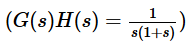is

Test: Control Systems - 1 - Question 3

### The transfer function G(s) of a PID controller is

Detailed Solution for Test: Control Systems - 1 - Question 3

Explanation : Simulation of a closed-loop system with proportional control. The process transfer function is P(s) = 1/(s + 1)3.

Test: Control Systems - 1 - Question 4

The industrial controller having the best steady - state accuracy is

Test: Control Systems - 1 - Question 5

consider the following statements :
1. The effect of feedback is to reduce the system error
2. Feedback increases the gain of the system in one frequency range but decreases in another.
3. Feedback can cause a system that is originally stable to become unstable
Which of these statements are correct ?

Test: Control Systems - 1 - Question 6

The intersection of asymptotes of root -loci of a  system with open loop transfer function is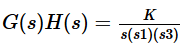Test: Control Systems - 1 - Question 7

The open-loop transfer function of unity feedback control system is  The system is stable is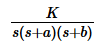0 < K < a/b (a+b)

Test: Control Systems - 1 - Question 8

Which one of the following characteristic equations of result in the stable operation of the feedback system ?

Test: Control Systems - 1 - Question 9

Consider the following statements: Routh-Hurwitz critierion gives
1. aboslute stability
2. the number of roots lying on the right half of the s-plane
3. the gainmargin and phase margin
Which of these statements are correct ?

Detailed Solution for Test: Control Systems - 1 - Question 9

Explanation : Routh-Hurwitz criterion gives absolute stability and number of roots lying on the right half of the s-plane.

Test: Control Systems - 1 - Question 10

Consider the following statements: A proportional plus derivative controller
1. has high sensitivity
2. increases the stability of the system
Which of these statements are correct ?

Test: Control Systems - 1 - Question 11

Open loop transfer function of a system having one zero with a positive real value is called

Test: Control Systems - 1 - Question 12

The given characteristic polynomial s4 + s3 + 2s2 + 2s + 3 =0 has

Test: Control Systems - 1 - Question 13

A control system has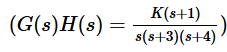Root locus of the system can lie on the real axis

Test: Control Systems - 1 - Question 14

The forward path transfer function of a unity feedback system is given by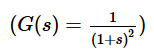What is the phase margin for this system ?

Test: Control Systems - 1 - Question 15

Which one of the following statement is correct ? The effects of phase lead compensator on gain crossover frequency (wcg) and the bandwidth (BW) are

Test: Control Systems - 1 - Question 16

What is the unit step response of a unity feedback control system having forward path transfer function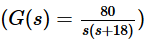Detailed Solution for Test: Control Systems - 1 - Question 16

The open loop transfer function is first converted into the closed loop as unity feedback is used and then value of damping factor is calculated.

Test: Control Systems - 1 - Question 17

An open loop system has a transfer function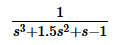it is converted into a closed loop system by providing a negative feedback having transfer function 20 (s+ 1). Which one of the following is correct ? The open loop and closed loop systems are, respectively

Test: Control Systems - 1 - Question 18

With regard to the filtering property, the lead compensator and the lag compensator are, respectively.

Test: Control Systems - 1 - Question 19

For the minimum phase system to be stable:

Test: Control Systems - 1 - Question 20

For the given system, how can be steady state error produced by step disturbance be reduced ?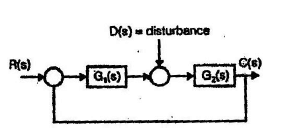Test: Control Systems - 1 - Question 21

If the gain of the open loop system is doubled, the gain margin of the system is

Test: Control Systems - 1 - Question 22

Which one of the following is not a property of root loci ?

Test: Control Systems - 1 - Question 23

Match List-I (System G(s) with List-II (Nature of response) and select the correct answer using the code given below the lists: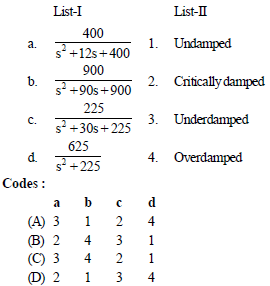Test: Control Systems - 1 - Question 24

Given a unity feedback system with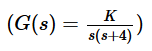what is the value of K for a damping ratio of 0.5 ?

Test: Control Systems - 1 - Question 25

What is the steady state error for a unity feedback control system having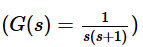due to unit ramp input ?

## Electrical Engineering SSC JE (Technical)

2 videos|75 docs|40 tests
Information about Test: Control Systems - 1 Page
In this test you can find the Exam questions for Test: Control Systems - 1 solved & explained in the simplest way possible. Besides giving Questions and answers for Test: Control Systems - 1, EduRev gives you an ample number of Online tests for practice

## Electrical Engineering SSC JE (Technical)

2 videos|75 docs|40 tests(Scan QR code)